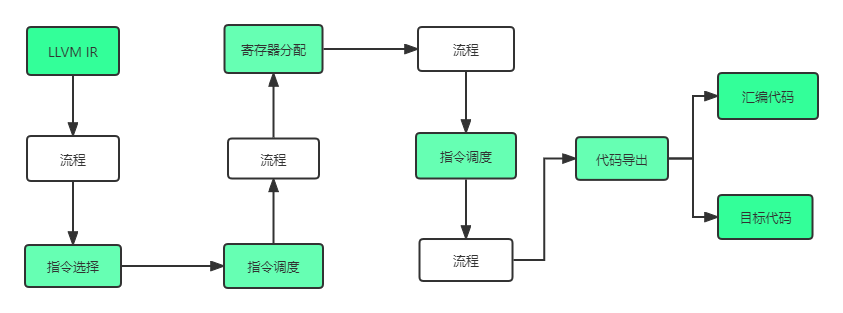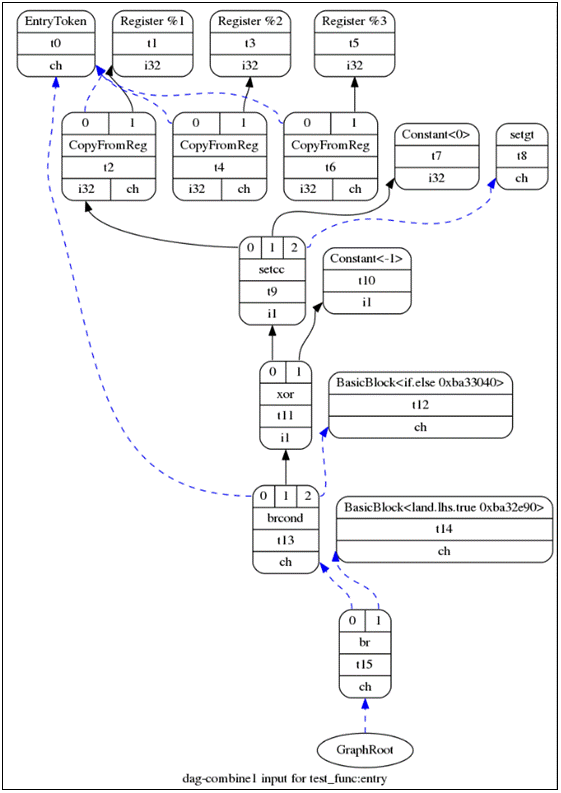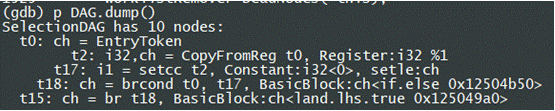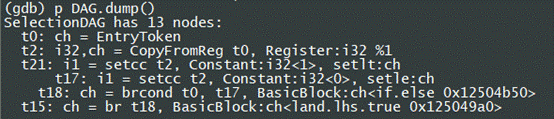# 针对LLVM的RISCV后端的的codesize优化 (BLT to BLEZ)

``````int test_func(int aa, int bb,int cc){
int jk = 7;
if(aa > 0 && bb > 0 && cc > 0){
jk = jk+ 20;
}
else{
jk = jk+4396;
}
return jk;
}``````

``````test_func:
blt     a0, a3, .LBB0_4
blt     a1, a3, .LBB0_4
blez    a2, .LBB0_4
ret
.LBB0_4:
lui     a0, 1
ret``````

``````test_func:
ble     a0,zero,.L5
ble     a1,zero,.L5
ble     a2,zero,.L5
li      a0,27
ret
.L5:
li      a0,4096
ret``````

## LLVM后端知识## 案例分析

``````./llc -march=riscv32 -mattr=+m ./test.ll -view-dag-combine1-dags
dot /tmp/dag.test_func-e3ff26.dot -Tpng -o ./a.png````````````./llc -march=riscv32 -mattr=+m ./test.ll -view-legalize-types-dags
dot /tmp/dag.test_func-e3ff26.dot -Tpng -o ./a.png``````Clang 的优化算法：TargetLowering.cpp line:3466 SimplifySetCC()

``````//line 3905
if (Cond == ISD::SETLE || Cond == ISD::SETULE) {
// X <= MAX --> true
if (C1 == MaxVal)
return DAG.getBoolConstant(true, dl, VT, OpVT);

// X <= C0 --> X < (C0 + 1)
if (!VT.isVector()) { // TODO: Support this for vectors.
APInt C = C1 + 1;
ISD::CondCode NewCC = (Cond == ISD::SETLE) ? ISD::SETLT : ISD::SETULT;
if ((DCI.isBeforeLegalizeOps() ||
isCondCodeLegal(NewCC, VT.getSimpleVT())) &&
(!N1C->isOpaque() || (C.getBitWidth() <= 64 &&
isLegalICmpImmediate(C.getSExtValue())))) {
return DAG.getSetCC(dl, VT, N0,
DAG.getConstant(C, dl, N1.getValueType()),
NewCC);
}
}
}``````

## 基于目标相关代码的优化

``````// line 2245
case ISD::SETCC: {
// (setcc X, 1, setne) -> (setcc X, 0, seteq) if we can prove X is 0/1.
// Comparing with 0 may allow us to fold into bnez/beqz.
SDValue LHS = N->getOperand(0);
SDValue RHS = N->getOperand(1);
if (LHS.getValueType().isScalableVector())
break;
auto CC = cast<CondCodeSDNode>(N->getOperand(2))->get();
if (isOneConstant(RHS) && ISD::isIntEqualitySetCC(CC) &&
SDLoc DL(N);
SDValue Zero = DAG.getConstant(0, DL, LHS.getValueType());
CC = ISD::getSetCCInverse(CC, LHS.getValueType());
return DAG.getSetCC(DL, N->getValueType(0), LHS, Zero, CC);
}
// (setcc X, 1, setlt/setgt) -> (setcc X, 0, setle/setge) if we can prove X is 0/1.
// Comparing with 0 may allow us to fold into blez/bgez.
if ((ISD::isSignedIntSetCC(CC) || ISD::isUnsignedIntSetCC(CC)) && isOneConstant(RHS)){
if (CC == ISD::SETGE || CC == ISD::SETUGE || CC == ISD::SETLE || CC == ISD::SETULE)
break;
unsigned OperationCC = CC;
SDLoc DL(N);
SDValue Zero = DAG.getConstant(0, DL, LHS.getValueType());
OperationCC ^= 1;
CC = ISD::CondCode(OperationCC);
//CC = ISD::getSetCCInverse(CC, LHS.getValueType());
return DAG.getSetCC(DL, N->getValueType(0), LHS, Zero, CC);
}
break;
}``````

## 基于目标无关代码的优化

``````SDValue DAGCombiner::combine(SDNode *N) {
SDValue RV;
DisableGenericCombines =false;
bool targetIsRISCV = false;
bool optLevelIsDefault = false;
const char* targetStr = DAG.getSubtarget().getTargetTriple().getTriple().c_str();
if (strncmp("riscv",targetStr,5)==0)
targetIsRISCV = true;
if (OptLevel == CodeGenOpt::Default)
optLevelIsDefault = true;
if (targetIsRISCV && optLevelIsDefault){
if (N->getOpcode() == ISD::SETCC){
auto CC = cast<CondCodeSDNode>(N->getOperand(2))->get();
if ((CC == ISD::SETGE || CC == ISD::SETUGE || CC == ISD::SETLE || CC == ISD::SETULE) && isNullFPConstant(N->getOperand(1))){
DisableGenericCombines = true;
}
}
}
...
}``````

## 效果

``````test_func:
blt     a0, a3, .LBB0_4
blt     a1, a3, .LBB0_4
blez    a2, .LBB0_4
ret
.LBB0_4:
lui     a0, 1
ret``````

``````test_func:
blez    a0, .LBB0_4
blez    a1, .LBB0_4